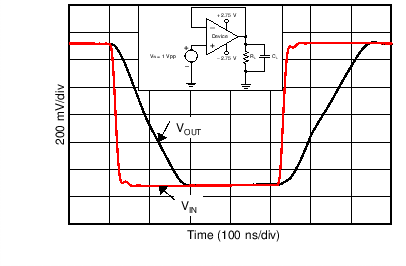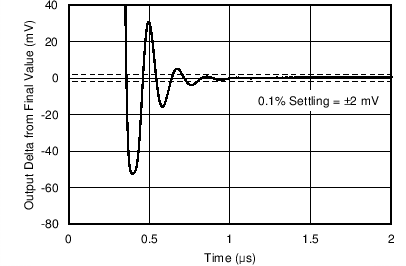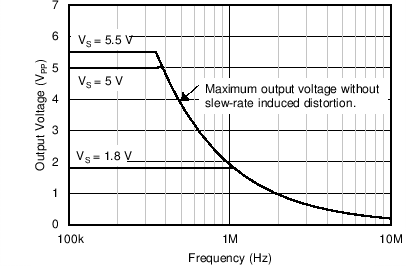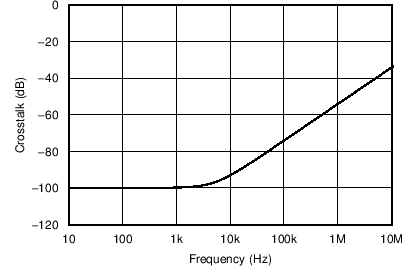SBOS703F April   2014  – October 2016

PRODUCTION DATA.

1. Features
2. Applications
3. Description
4. Revision History
5. Pin Configuration and Functions
6. Specifications
7. Detailed Description
1. 7.1 Overview
2. 7.2 Functional Block Diagram
3. 7.3 Feature Description
4. 7.4 Device Functional Modes
8. Application and Implementation
1. 8.1 Application Information
2. 8.2 Typical Application
9. Power Supply Recommendations
10. 10Layout
11. 11Device and Documentation Support
12. 12Mechanical, Packaging, and Orderable Information
13. 11Mechanical, Packaging, and Orderable Information

• PW|14
• D|14
• PW|14
• D|14

## 6 Specifications

### 6.1 Absolute Maximum Ratings

over operating free-air temperature (unless otherwise noted)(1)
MIN MAX UNIT
Supply voltage 7 V
Signal input pins Voltage(2) Common-mode (V–) – 0.5 (V+) + 0.5 V
Differential (V+) – (V–) + 0.2 V
Current(2) –10 10 mA
Output short-circuit(3) Continuous
TA Operating temperature –55 150 °C
TJ Junction temperature 150 °C
Tstg Storage temperature –65 150 °C
(1) Stresses beyond those listed under Absolute Maximum Ratings may cause permanent damage to the device. These are stress ratings only, which do not imply functional operation of the device at these or any other conditions beyond those indicated under Recommended Operating Conditions. Exposure to absolute-maximum-rated conditions for extended periods may affect device reliability.
(2) Input pins are diode-clamped to the power-supply rails. Current limit input signals that can swing more than 0.5 V beyond the supply rails to 10 mA or less.
(3) Short-circuit to ground, one amplifier per package.

### 6.2 ESD Ratings

over operating free-air temperature range (unless otherwise noted).
VALUE UNIT
V(ESD) Electrostatic discharge Human body model (HBM), per ANSI/ESDA/JEDEC JS-001(1) ±4000 V
Charged device model (CDM), per JEDEC specification JESD22-C101(2) ±1500
(1) JEDEC document JEP155 states that 500-V HBM allows safe manufacturing with a standard ESD control process.
(2) JEDEC document JEP157 states that 250-V CDM allows safe manufacturing with a standard ESD control process.

### 6.3 Recommended Operating Conditions

over operating free-air temperature range (unless otherwise noted).
MIN MAX UNIT
VS Supply voltage 1.8 5.5 V
Specified temperature –40 125 °C

### 6.4 Thermal Information: OPA316

THERMAL METRIC(1) OPA316 UNIT
DBV (SOT23) DCK (SC70)
5 PINS 5 PINS
RθJA Junction-to-ambient thermal resistance(2) 221.7 263.3 °C/W
RθJC(top) Junction-to-case(top) thermal resistance(3) 144.7 75.5 °C/W
RθJB Junction-to-board thermal resistance(4) 49.7 51 °C/W
ψJT Junction-to-top characterization parameter(5) 26.1 1 °C/W
ψJB Junction-to-board characterization parameter(6) 49 50.3 °C/W
RθJC(bot) Junction-to-case(bottom) thermal resistance(7) N/A N/A °C/W
(2) The junction-to-ambient thermal resistance under natural convection is obtained in a simulation on a JEDEC-standard, high-K board, as specified in JESD51-7, in an environment described in JESD51-2a.
(3) The junction-to-case (top) thermal resistance is obtained by simulating a cold plate test on the package top. No specific JEDEC-standard test exists, but a close description can be found in the ANSI SEMI standard G30-88.
(4) The junction-to-board thermal resistance is obtained by simulating in an environment with a ring cold plate fixture to control the PCB temperature, as described in JESD51-8.
(5) The junction-to-top characterization parameter, ψJT, estimates the junction temperature of a device in a real system and is extracted from the simulation data for obtaining RθJA, using a procedure described in JESD51-2a (sections 6 and 7).
(6) The junction-to-board characterization parameter, ψJB, estimates the junction temperature of a device in a real system and is extracted from the simulation data for obtaining RθJA, using a procedure described in JESD51-2a (sections 6 and 7).
(7) The junction-to-case (bottom) thermal resistance is obtained by simulating a cold plate test on the exposed (power) pad. No specific JEDEC standard test exists, but a close description can be found in the ANSI SEMI standard G30-88.

### 6.5 Thermal Information: OPA2316

THERMAL METRIC(1) OPA2316 UNIT
D (SO) DGK (MSOP) DRG (DFN)
8 PINS 8 PINS 8 PINS
RθJA Junction-to-ambient thermal resistance(2) 127.2 186.6 56.3 °C/W
RθJC(top) Junction-to-case(top) thermal resistance(3) 71.6 78.8 72.2 °C/W
RθJB Junction-to-board thermal resistance(4) 68.2 107.9 31 °C/W
ψJT Junction-to-top characterization parameter(5) 22 15.5 2.3 °C/W
ψJB Junction-to-board characterization parameter(6) 67.6 106.3 21.2 °C/W
RθJC(bot) Junction-to-case(bottom) thermal resistance(7) N/A N/A 10.9 °C/W
(2) The junction-to-ambient thermal resistance under natural convection is obtained in a simulation on a JEDEC-standard, high-K board, as specified in JESD51-7, in an environment described in JESD51-2a.
(3) The junction-to-case (top) thermal resistance is obtained by simulating a cold plate test on the package top. No specific JEDEC-standard test exists, but a close description can be found in the ANSI SEMI standard G30-88.
(4) The junction-to-board thermal resistance is obtained by simulating in an environment with a ring cold plate fixture to control the PCB temperature, as described in JESD51-8.
(5) The junction-to-top characterization parameter, ψJT, estimates the junction temperature of a device in a real system and is extracted from the simulation data for obtaining RθJA, using a procedure described in JESD51-2a (sections 6 and 7).
(6) The junction-to-board characterization parameter, ψJB, estimates the junction temperature of a device in a real system and is extracted from the simulation data for obtaining RθJA, using a procedure described in JESD51-2a (sections 6 and 7).
(7) The junction-to-case (bottom) thermal resistance is obtained by simulating a cold plate test on the exposed (power) pad. No specific JEDEC standard test exists, but a close description can be found in the ANSI SEMI standard G30-88.

### 6.6 Thermal Information: OPA2316S

THERMAL METRIC(1) OPA2316S UNIT
DGS (MSOP) QFN (RUG)
10 PINS 10 PINS
RθJA Junction-to-ambient thermal resistance(2) 189.6 158 °C/W
RθJC(top) Junction-to-case(top) thermal resistance(3) 73.9 52 °C/W
RθJB Junction-to-board thermal resistance(4) 110.7 88 °C/W
ψJT Junction-to-top characterization parameter(5) 13.4 1 °C/W
ψJB Junction-to-board characterization parameter(6) 109.1 87 °C/W
RθJC(bot) Junction-to-case(bottom) thermal resistance(7) N/A N/A °C/W
(2) The junction-to-ambient thermal resistance under natural convection is obtained in a simulation on a JEDEC-standard, high-K board, as specified in JESD51-7, in an environment described in JESD51-2a.
(3) The junction-to-case (top) thermal resistance is obtained by simulating a cold plate test on the package top. No specific JEDEC-standard test exists, but a close description can be found in the ANSI SEMI standard G30-88.
(4) The junction-to-board thermal resistance is obtained by simulating in an environment with a ring cold plate fixture to control the PCB temperature, as described in JESD51-8.
(5) The junction-to-top characterization parameter, ψJT, estimates the junction temperature of a device in a real system and is extracted from the simulation data for obtaining RθJA, using a procedure described in JESD51-2a (sections 6 and 7).
(6) The junction-to-board characterization parameter, ψJB, estimates the junction temperature of a device in a real system and is extracted from the simulation data for obtaining RθJA, using a procedure described in JESD51-2a (sections 6 and 7).
(7) The junction-to-case (bottom) thermal resistance is obtained by simulating a cold plate test on the exposed (power) pad. No specific JEDEC standard test exists, but a close description can be found in the ANSI SEMI standard G30-88.

### 6.7 Thermal Information: OPA4316

THERMAL METRIC(1) OPA4316 UNIT
PW (TSSOP) D (SOIC)
14 PINS 14 PINS
RθJA Junction-to-ambient thermal resistance(2) 117.2 87.0 °C/W
RθJC(top) Junction-to-case(top) thermal resistance(3) 46.2 44.4 °C/W
RθJB Junction-to-board thermal resistance(4) 58.9 41.7 °C/W
ψJT Junction-to-top characterization parameter(5) 4.9 11.6 °C/W
ψJB Junction-to-board characterization parameter(6) 58.3 41.4 °C/W
RθJC(bot) Junction-to-case(bottom) thermal resistance(7) N/A N/A °C/W
(2) The junction-to-ambient thermal resistance under natural convection is obtained in a simulation on a JEDEC-standard, high-K board, as specified in JESD51-7, in an environment described in JESD51-2a.
(3) The junction-to-case (top) thermal resistance is obtained by simulating a cold plate test on the package top. No specific JEDEC-standard test exists, but a close description can be found in the ANSI SEMI standard G30-88.
(4) The junction-to-board thermal resistance is obtained by simulating in an environment with a ring cold plate fixture to control the PCB temperature, as described in JESD51-8.
(5) The junction-to-top characterization parameter, ψJT, estimates the junction temperature of a device in a real system and is extracted from the simulation data for obtaining RθJA, using a procedure described in JESD51-2a (sections 6 and 7).
(6) The junction-to-board characterization parameter, ψJB, estimates the junction temperature of a device in a real system and is extracted from the simulation data for obtaining RθJA, using a procedure described in JESD51-2a (sections 6 and 7).
(7) The junction-to-case (bottom) thermal resistance is obtained by simulating a cold plate test on the exposed (power) pad. No specific JEDEC standard test exists, but a close description can be found in the ANSI SEMI standard G30-88.

### 6.8 Electrical Characteristics

VS (total supply voltage) = (V+) – (V–) = 1.8 V to 5.5 V.
at TA = 25°C, RL = 10 kΩ connected to VS / 2, VCM = VS / 2, and VOUT = VS / 2, unless otherwise noted.
PARAMETER TEST CONDITIONS MIN TYP MAX UNIT
OFFSET VOLTAGE
VOS Input offset voltage VS = 5 V ±0.5 ±2.5 mV
VS = 5 V, TA = –40°C to 125°C ±3.5 mV
dVOS/dT Drift VS = 5 V, TA = –40°C to 125°C ±2 ±10 μV/°C
PSRR vs power supply VS = 1.8 V – 5.5 V, VCM = (V–) ±30 ±150 µV/V
VS = 1.8 V – 5.5 V, VCM = (V–), TA = –40°C to 125°C ±250 µV/V
Channel separation, dc At dc 10 µV/V
INPUT VOLTAGE RANGE
VCM Common-mode voltage VS = 1.8 V to 2.5 V (V–) – 0.2 (V+) V
VS = 2.5 V to 5.5 V (V–) – 0.2 (V+) + 0.2 V
CMRR Common-mode rejection ratio VS = 1.8 V, (V–) – 0.2 V < VCM < (V+) – 1.4 V,
TA= –40°C to 125°C
70 86 dB
VS = 5.5 V, (V–) – 0.2 V < VCM < (V+) – 1.4 V,
TA= –40°C to 125°C
76 90 dB
VS = 1.8 V, VCM = –0.2 V to 1.8 V,
TA= –40°C to 125°C
57 72 dB
VS = 5.5 V, VCM = –0.2 V to 5.7 V,
TA= –40°C to 125°C
65 80 dB
INPUT BIAS CURRENT
IB Input bias current ±5 ±15 pA
TA= –40°C to 125°C ±15 nA
IOS Input offset current ±2 ±15 pA
TA= –40°C to 125°C ±8 nA
NOISE
En Input voltage noise (peak-to-peak) VS = 5 V, f = 0.1 Hz to 10 Hz 3 μVPP
en Input voltage noise density VS = 5 V, f = 1 kHz 11 nV/√Hz
in Input current noise density f = 1 kHz 1.3 fA/√Hz
INPUT IMPEDANCE
ZID Differential 2 || 2 1016Ω || pF
ZIC Common-mode 2 || 4 1011Ω || pF
OPEN-LOOP GAIN
AOL Open-loop voltage gain VS = 1.8 V, (V–) + 0.04 V < VO < (V+) – 0.04 V,
RL = 10 kΩ
94 100 dB
VS = 5.5 V, (V–) + 0.05 V < VO < (V+) – 0.05 V,
RL = 10 kΩ
104 110 dB
VS = 1.8 V, (V–) + 0.1 V < VO < (V+) – 0.1 V,
RL = 2 kΩ
90 96 dB
VS = 5.5 V, (V–) + 0.15 V < VO < (V+) – 0.15 V,
RL = 2 kΩ
100 106 dB
VS = 5.5 V, (V–) + 0.05 V < VO < (V+) – 0.05 V,
RL = 10 kΩ, TA= –40°C to 125°C
86 dB
VS = 5.5 V, (V–) + 0.15 V < VO < (V+) – 0.15 V,
RL = 2 kΩ, TA= –40°C to 125°C
84 dB
FREQUENCY RESPONSE
GBP Gain bandwidth product VS = 5 V, G = +1 10 MHz
φm Phase margin VS = 5 V, G = +1 60 Degrees
SR Slew rate VS = 5 V, G = +1 6 V/μs
tS Settling time To 0.1%, VS = 5 V, 2-V step , G = +1, CL = 100 pF 1 μs
To 0.01%, VS = 5 V, 2-V step , G = +1, CL = 100 pF 1.66 μs
tOR Overload recovery time VS = 5 V, VIN  × gain = VS 0.3 μs
THD + N Total harmonic distortion + noise(1) VS = 5 V, VO = 0.5 VRMS, G = +1, f = 1 kHz 0.0008%
OUTPUT
VO Voltage output swing from supply rails VS = 1.8 V, RL = 10 kΩ, TA= –40°C to 125°C 15 mV
VS = 5.5 V, RL = 10 kΩ, TA= –40°C to 125°C 30 mV
VS = 1.8 V, RL = 2 kΩ, TA= –40°C to 125°C 60 mV
VS = 5.5 V, RL = 2 kΩ, TA= –40°C to 125°C 120 mV
ISC Short-circuit current VS = 5 V ±50 mA
ZO Open-loop output impedance VS = 5 V, f = 10 MHz 250 Ω
POWER SUPPLY
VS Specified voltage 1.8 5.5 V
IQ Quiescent current per amplifier VS = 5 V, IO = 0 mA, TA= –40°C to 125°C 400 500 µA
Power-on time VS = 0 V to 5.5 V 200 µs
SHUTDOWN (VS = 1.8 V to 5.5 V)(2)
IQSD Quiescent current, per device All amplifiers disabled, SHDN = VS– 0.01 1 µA
One amplifier disabled (OPA2316S) 345 µA
VIH High voltage (enabled) Amplifier enabled (V+) – 0.5 V
VIL Low voltage (disabled) Amplifier disabled (V–) + 0.2 V
tON Amplifier enable time(3) Full shutdown, G = 1, VOUT = 0.9 × VS / 2(4) 13 µs
Partial shutdown, G = 1, VOUT = 0.9 × VS / 2(4) 10 µs
tOFF Amplifier disable time(3) G = 1, VOUT = 0.1 × VS / 2 5 µs
SHDN pin input bias current (per pin) VIH = 5 V 3.5 pA
VIL = 0 V 2.5 pA
TEMPERATURE
Specified temperature –40 125 °C
TA Operating temperature –55 150 °C
Tstg Storage temperature –65 150 °C
(1) Third-order filter; bandwidth = 80 kHz at –3 dB.
(2) Ensured by design and characterization; not production tested.
(3) Enable time (tON) and disable time (tOFF) are defined as the time interval between the 50% point of the signal applied to the SHDN pin and the point at which the output voltage reaches the 10% (disable) or 90% (enable) level.
(4) Full shutdown refers to the dual OPA2316S having both channels A and B disabled (SHDN_A = SHDN_B = VS–). For partial shutdown, only one SHDN pin is exercised; in partial mode, the internal biasing and oscillator remain operational and the enable time is shorter.

### 6.9 Typical Characteristics

at TA = 25°C, VS = 5.5 V, RL = 10 kΩ connected to VS / 2, VCM = VS / 2, and VOUT = VS / 2, unless otherwise noted.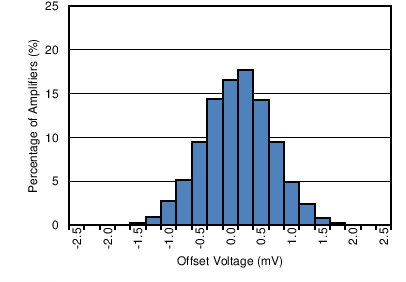Distribution taken from 12551 amplifiers
Figure 1. Offset Voltage Production Distribution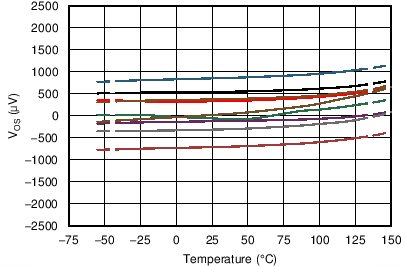9 typical units shown
Figure 3. Offset Voltage vs Temperature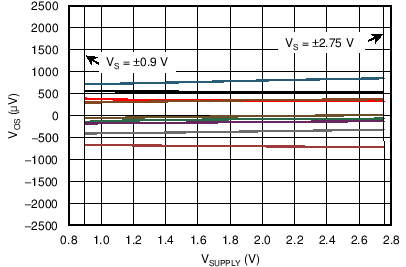V+ = 0.9 V to 2.75 V, V– = –0.9 V to –2.75 V, 9 typical units shown
Figure 5. Offset Voltage vs Power Supply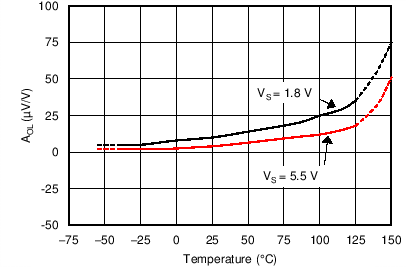RL = 10 kΩ
Figure 7. Open-Loop Gain vs Temperature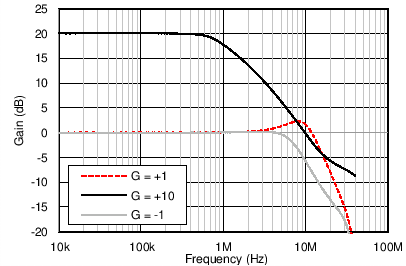Figure 9. Closed-Loop Gain vs FrequencyV+ = 2.75 V, V– = –2.75 V
Figure 11. Output Voltage Swing vs Output Current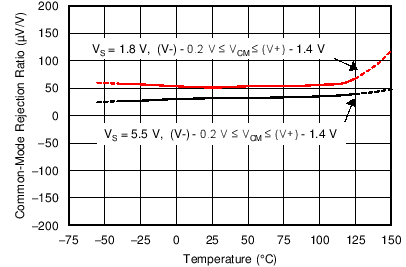Figure 13. CMRR vs Temperature (Narrow Range)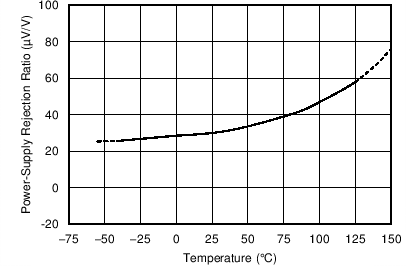Figure 15. PSRR vs Temperature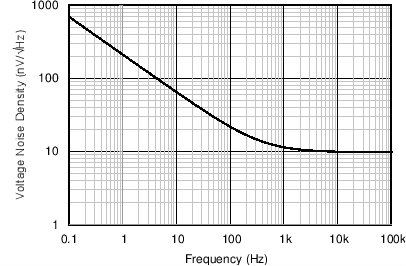Figure 17. Input Voltage Noise Spectral Density vs Frequency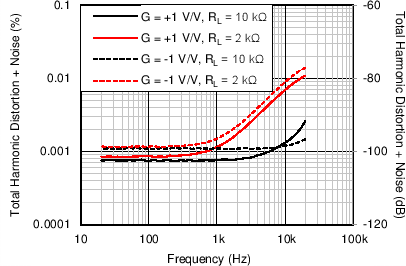BW = 80 kHz, VOUT = 0.5 VRMS
Figure 19. THD + N vs Frequency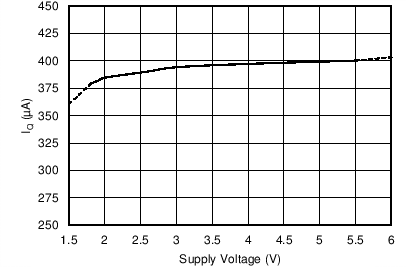Figure 21. Quiescent Current vs Supply Voltage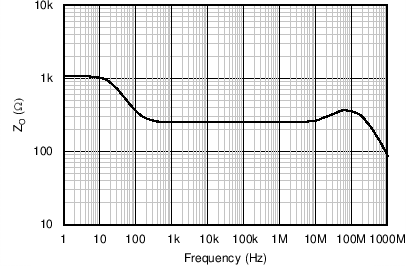Figure 23. Open-Loop Output Impedance vs Frequency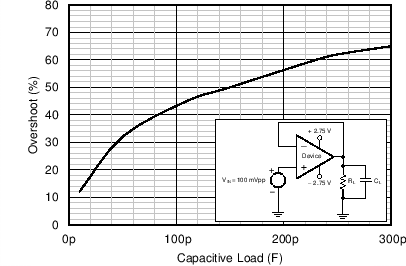V+ = 2.75 V, V– = –2.75 V , G = +1 V/V, RL = 1 kΩ
Figure 25. Small-Signal Overshoot vs Load Capacitance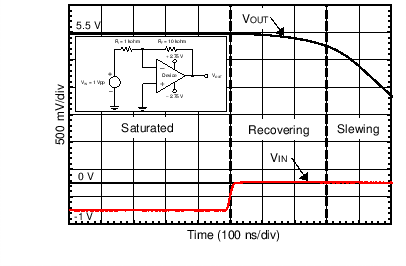V+ = 2.75 V, V– = –2.75 V , G = –10 V/V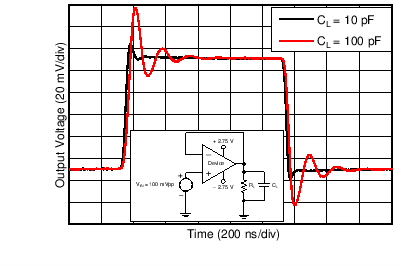V+ = 2.75 V, V– = –2.75 V, G = +1 V/V
Figure 29. Small-Signal Step Response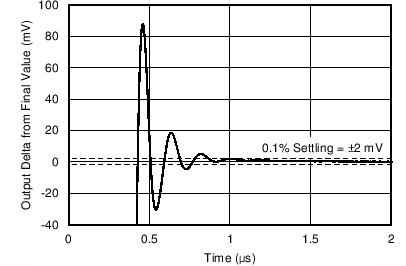CL = 100 pF, G = +1 V/V
Figure 31. Positive Large-Signal Settling Time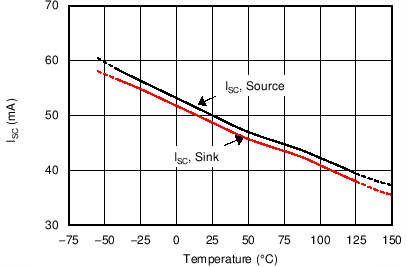Figure 33. Short-Circuit Current vs Temperature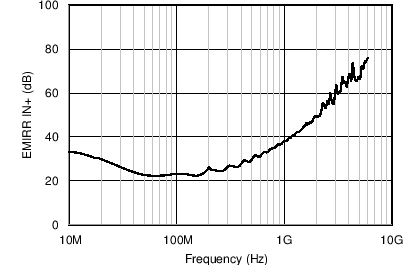PRF = –10 dBm
Figure 35. Electromagnetic Interference Rejection Ratio
Referred to Noninverting Input (EMIRR IN+) vs Frequency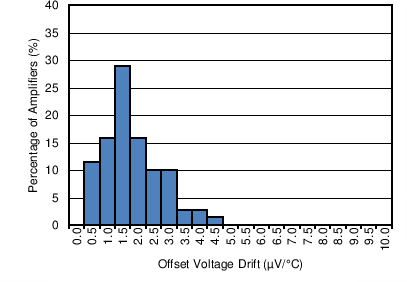TA = –40°C to +125°C, Distribution taken from 70 amplifiers
Figure 2. Offset Voltage Drift Distribution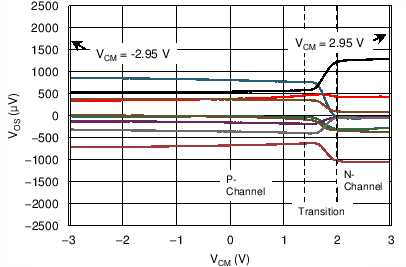V+ = 2.75 V, V– = –2.75 V, 9 typical units shown
Figure 4. Offset Voltage vs Common-Mode Voltage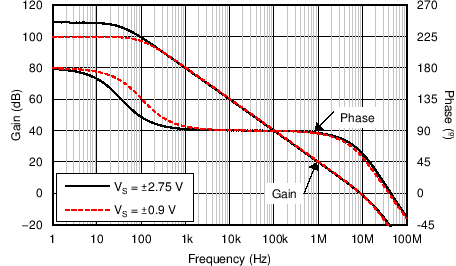VCM < (V+) – 1.4 V
Figure 6. Open-Loop Gain and Phase vs Frequency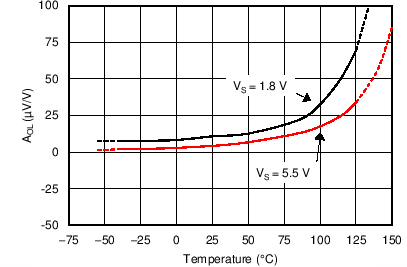RL = 2 kΩ
Figure 8. Open-Loop Gain vs Temperature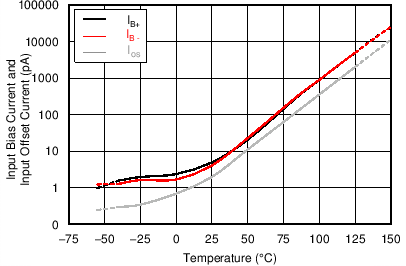Figure 10. Input Bias and Offset Current vs Temperature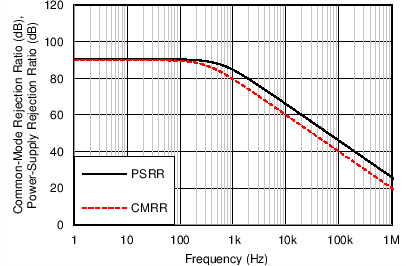Figure 12. CMRR and PSRR vs Frequency
(Referred to Input)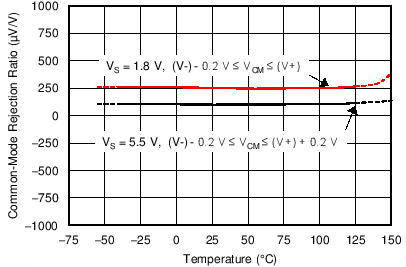Figure 14. CMRR vs Temperature (Wide Range)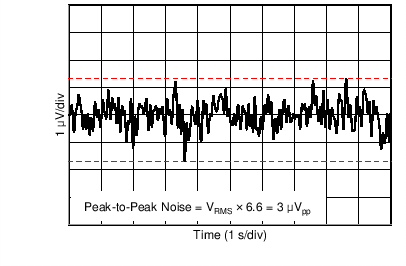Figure 16. 0.1-Hz to 10-Hz Input Voltage Noise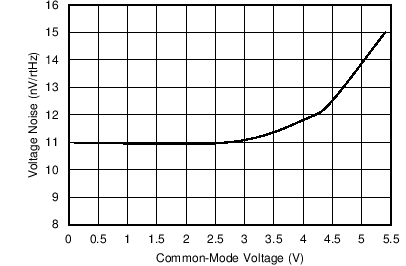ƒ = 1 kHz
Figure 18. Input Voltage Noise vs Common-Mode Voltage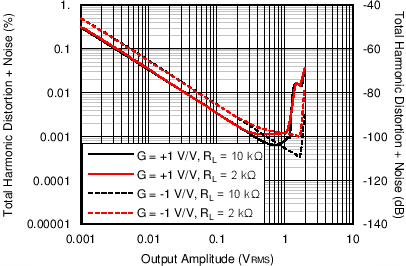ƒ = 1 kHz, BW = 80 kHz
Figure 20. THD + N vs Amplitude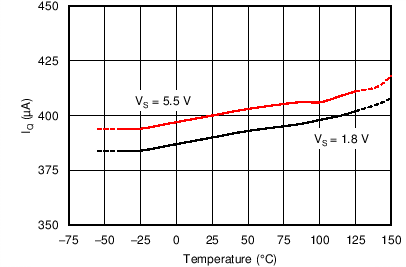Figure 22. Quiescent Current vs Temperature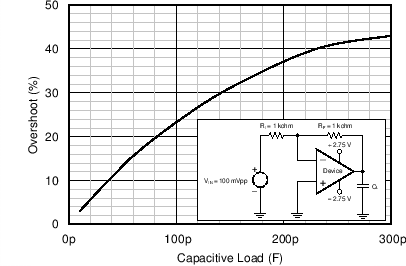V+ = 2.75 V, V– = –2.75 V, G = –1 V/V
Figure 24. Small-Signal Overshoot vs Load Capacitance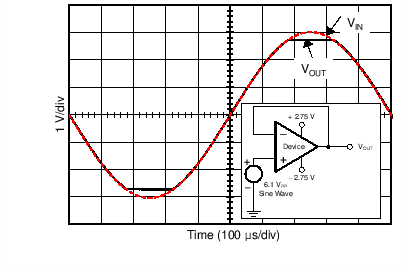V+ = 2.75 V, V– = –2.75 V
Figure 26. No Phase Reversal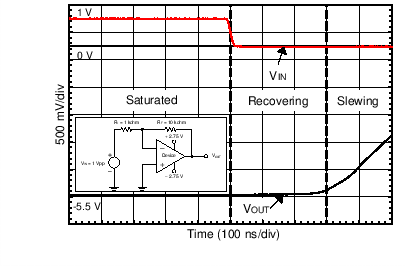V+ = 2.75 V, V– = –2.75 V, G = –10 V/V